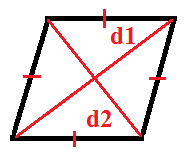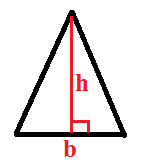# Area Calculator for Rectangle, Square, Trapezium, Circle, Parallelogram

## Area of Square Calculator## Area of parallelogram Calculator## Area of Rhombus Calculator## Area of General Triangle Calculator## Area of ellipse Calculator## Area of Regular Polygons Calculator## Area of Regular Polygons Calculator## Area of Sector Circle Calculator°(Degree)

## Area of Circle Calculator## Area of Semi Circle Calculator## How to use Area calculator?

1. Select the object for which you want to calculate the area.
2. For ease, we have provided figures above each calculator.
3. Now, input the value for the dimension of an object like length, width, height, radius, etc according to the calculator.
4. Choose the unit for different dimensions from the drop-down list.
5. Click on calculate button to get the area of a different object in a different unit.
6. Enjoy accurate value and rapid calculation in the Area calculator.

Area Calculator is the tool that helps you to calculate the area of different objects like a triangle, rectangles, squares, ellipses, sectors of circles, trapeziums, parallelograms, etc. It makes it easy to calculate rapidly when we have dimensions of different objects with different units.

Area calculator can be used by students, teachers, or general people who require to calculate the area of different geometrical shapes for different purposes.

## 1) Rectangle

Definition:

Rectangle can be defined as a shape with four straight sides and four angles of 900 (right angle) two sides are longer than other two sides.

Formula for Perimeter: 2(a+b)

Formula to calculate Area of Rectangle (A) = a x b

How to calculate area of rectangle?

Example:

Let us consider a rectangle with its length(a) = 8cm and its breadth(b) = 4cm, its area can be calculated by

Solution,

We know that, area or a rectangle = a x b

= 8cm x 4cm

.’. Area of the given rectangle   = 32cm2

## 2) Square

Definition:

Square can be defined as a closed two-dimensional shape with 4 equal sides. A square is a quadrilateral.

Formula for Perimeter: 4a

Formula to calculate Area of Square (A): a2

How to calculate area of square?

Example:

Let us consider a square with side(a) = 4cm, its area can be calculated by

Solution,

We know that area of square = a2

= (4cm)2

.’. Area of the given square =   16cm2

## 3) Parallelogram

Definition:

Parallelogram can be defined as the quadrilateral whose opposites sides are parallel and alternate angles are equal.

Formula for Perimeter: 2(a+b)

Formula to calculate Area of parallelogram (A): b x h

How to calculate area of parallelogram?

Example:

Let us consider a parallelogram with length of one side(a) = 4cm, another side(b) = 7cm and height(h) = 3cm, its area can be calculated by

Solution,

We know that area of a parallelogram = b x h

= 7cm x 3cm

.’. Area of the given parallelogram = 21cm2

## 4) Rhombus

Definition:

Rhombus can be defined as a parallelogram whose all sides are equal to each other.

Formula for Perimeter: 4a

Formula to calculate Area of rhombus (A): (1/2).𝑑1.𝑑2

How to calculate area of rhombus?

Example:

Let us consider a rhombus with its sides(a) = 6cm, its diagonal (d1) = 8cm and another diagonal(d2) = 7cm, its area can be calculated be

Solution,

We know that area of a rhombus = (1/2).𝑑1.𝑑2

= (1/2)⋅8𝑐𝑚⋅7𝑐𝑚

.’. Area of the given rhombus = 28cm2

## 5) Trapezium

Definition:

Trapezium can be defined as a quadrilateral in which one pair of opposite sides are parallel to each other.

Formula for Perimeter: Sum of 4 sides

Formula to calculate Area of trapezium (A): (1/2) * h(𝑎+𝑏)

How to calculate area of trapezium?

Example:

Let us consider a trapezium with two parallel sides a and b, length of a is taken as 15cm and length of b is taken as 10cm and its height is 8cm, its area can be calculated by

Solution,

We know that area of a trapezium = (1/2).h.(𝑎+𝑏)

= (1/2)⋅8𝑐𝑚.(15𝑐𝑚+10𝑐𝑚)

.’. Area of the given trapezium = 100cm2

## 6) General triangle

Definition:

Triangle can be defined as a polygon made up of 3 line segment.

Formula for Perimeter: a+b+c

Formula to calculate Area of General Triangle (A): 1/2.𝑏.h

How to calculate area of a general triangle?

Example:

Let us consider a triangle with sides a, b and c having length of 6cm, 9cm and 4cm continuously and having a height(h) = 2cm, its area can be calculated by,

Solution,

We know that area of a triangle = (1/2) x 𝑏 x h

= (1/2)⋅9𝑐𝑚⋅2𝑐𝑚

.’. Area of the given triangle = 9cm2

## 7) Equilateral triangle

Definition:

It can be defined as a triangle whose all three sides are equal.

Formula for Perimeter: 3a

Formula to calculate Area of equilateral triangle (A):

How to calculate area of an equilateral triangle?

Example:

Let us consider an equilateral triangle with sides (a) = 7cm and height (h) = 5cm, its area can be calculated by

Solution,

We know that area of equilateral triangle =

= 0.43 x (7𝑐𝑚)2

.’. Area of the given equilateral triangle = 21.22 cm2

## 8) Right angled triangle

Definition:

Right angled triangle can be defined as a triangle having two any of its side perpendicular to each other.

Formula for Perimeter: b+h+d

Formula to calculate Area of right angled triangle (A): 1/2.𝑏.h

How to calculate area of a right-angled triangle?

Example:

Let us consider a right-angled triangle having base (b) = 3cm, height (h) = 5cm and the third line (d) = 7cm, its volume can be calculated by

Solution,

We know that area of a right angled triangle = 1/2 x 𝑏 x h

= 1/2 x 3𝑐𝑚 x5𝑐𝑚

.’. Area of the given right-angled triangle = 7.5 cm2

## 9) Isosceles Right Triangle.

Definition:

An isosceles right triangle can be defined as a triangle having its two sides equal and perpendicular to each other.

Formula for Perimeter: 2a+d

Formula to calculate Area of isosceles triangle (A): 1/2.𝑎2

How to calculate area of an isosceles right triangle?

Example:

Let us consider an isosceles right triangle having equal sides (a) = 8cm and another side (d) = 12cm, its area can be calculated by

Solution,

We know that area of isosceles right triangle = 1/2.𝑎2

= (1/2).82 𝑐𝑚2

.’. Area of the given isosceles right triangle = 32cm2

## 10) Regular Polygon

Definition:

Polygon can be defined as a simple closed figure made up of three or more-line segments is called polygon.

Formula for Perimeter: ns

Formula to calculate Area of Regular Polygon (A):: 𝑛.(1/2).𝑎.𝑠

where, n is number of sides

How to calculate area of a Regular polygon?

Example:

Let us consider a polygon having 5 sides (s) of length 5cm and a line perpendicular to each sides crossing the center (a) = 4cm, its area can be calculated by,

Solution,

We know that area of a polygon = 𝑛.(1/2).𝑎.𝑠

= 5 x (1/2) x 4𝑐𝑚 x 5𝑐𝑚

.’. Area of the given polygon = 50 cm2

## 11) Circle

Definition:

Circle can be defined as all points in the same plane that lie at an equal distance from a center point.

Formula for Perimeter: 2𝜋𝑟

Formula to calculate Area of Circle (A): 𝜋𝑟2

How to calculate area of a circle?

Example:

Let us consider a circle having radius(r) = 6cm, its area can be calculated by,

Solution,

We know that area of a circle = 𝜋𝑟2

= (22/7)*62 𝑐𝑚2

.’. Area of the given square = 113.14cm2

## 12) Semi-circle

Definition:

Half of circle is known as semicircle.

Formula for Perimeter: 𝜋𝑟+2𝑟

Formula to calculate Area of Semi Circle (A): 1/2 x 𝜋 x 𝑟2

How to calculate area of a semi-circle?

Example:

Let us consider a semicircle of radius(r) = 2.5cm, its area can be calculated by

Solution,

We know that area of a semicircle = 1/2 x 𝜋 x 𝑟2

= (1/2) x (22/7) x 2.52 𝑐𝑚2

.’. Area of the given semicircle = 9.82cm2

## 13) Sector of a circle

Definition:

The sector of a circle can be defined as a part taken from a circle, that part is made by two different radiuses making an angle (𝜃) Between them.

The formula for Perimeter: 𝑙+2𝜋

Formula to calculate Area of Sector of circle (A): (𝜃/360) x 2.𝜋𝑟.2

Formula for Where, i = 𝜃/360⋅2𝜋𝑟

How to calculate area of a sector of a circle?

Example:

Let us consider a circle with radius(r) = 5cm, angle between two radius (θ) = 450 , its area can be calculated by

Solution,

We know that area of sector of a circle = (𝜃/360) x 𝜋𝑟.2

= (45/360)*(22/7)*52 𝑐𝑚2

.’. Area of the given sector of circle = 9.82 cm2

## 14) Ellipse

Definition:

Ellipse can be defined as a circle which have been stretched or elongated in one of any direction to give it a shape of oval from circle.

Formula for Perimeter:  𝜋3𝑎+𝑏−𝑎+2𝑏+𝑏+2𝑎

Formula to calculate Area of ellipse (A): 𝜋𝑎𝑏

How to calculate area of the ellipse?

Example

Let us consider an ellipse with one of its axis (a) = 5cm and another axis (b) = 7cm, its area can be calculated by

Solution,

We know that area of ellipse = 𝜋𝑎𝑏

= 227⋅5𝑐𝑚⋅7𝑐𝑚

.’. Area of the given ellipse = 110cm2

Use Also

Volume Calculator for Cylinder, Sphere, Cone, Frustum, Cuboid, Pyramid

Volume of Soil in Excavation Calculator | Cutting and Filling

Rate Analysis of Plaster works Calculator

Sharing Is Caring: